• 对于python基础运算而言，想必大家都很熟悉，运算按种类可分为算数运算、比较运算、逻辑运算、赋值运算、成员运算、身份运算、位运算。 一、算数运算 以下假设变量：a=10，b=20 二、比较运算 以下假设变量...
对于python基础的运算而言，想必大家都很熟悉，运算按种类可分为算数运算、比较运算、逻辑运算、赋值运算、成员运算、身份运算、位运算。

一、算数运算

以下假设变量：a=10，b=20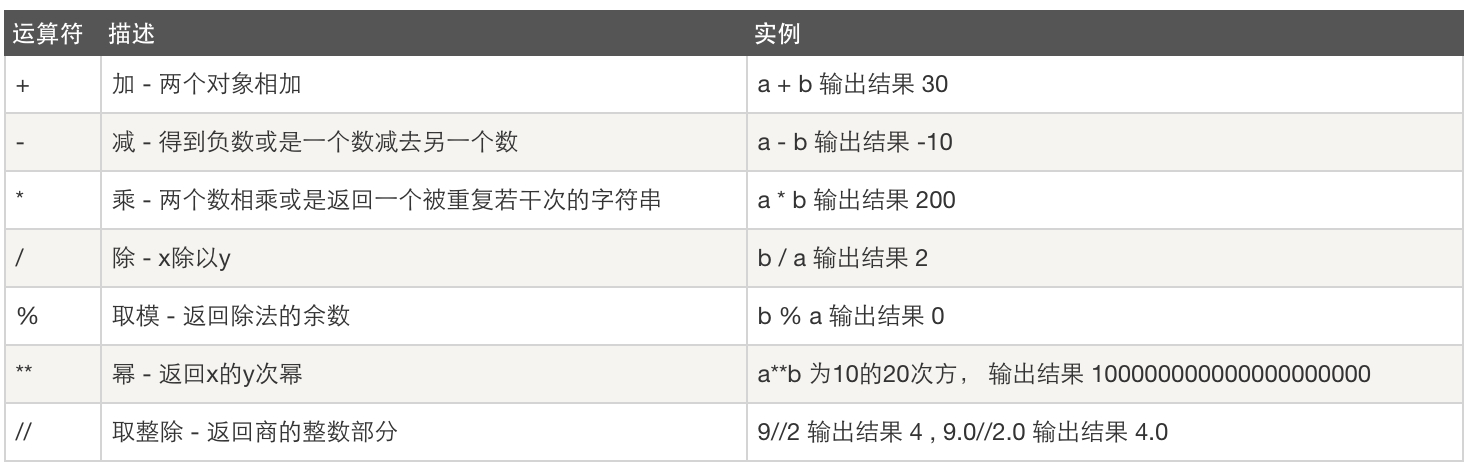二、比较运算

以下假设变量：a=10，b=20，注：<>不等于在python2中使用，python3中不等于为 !=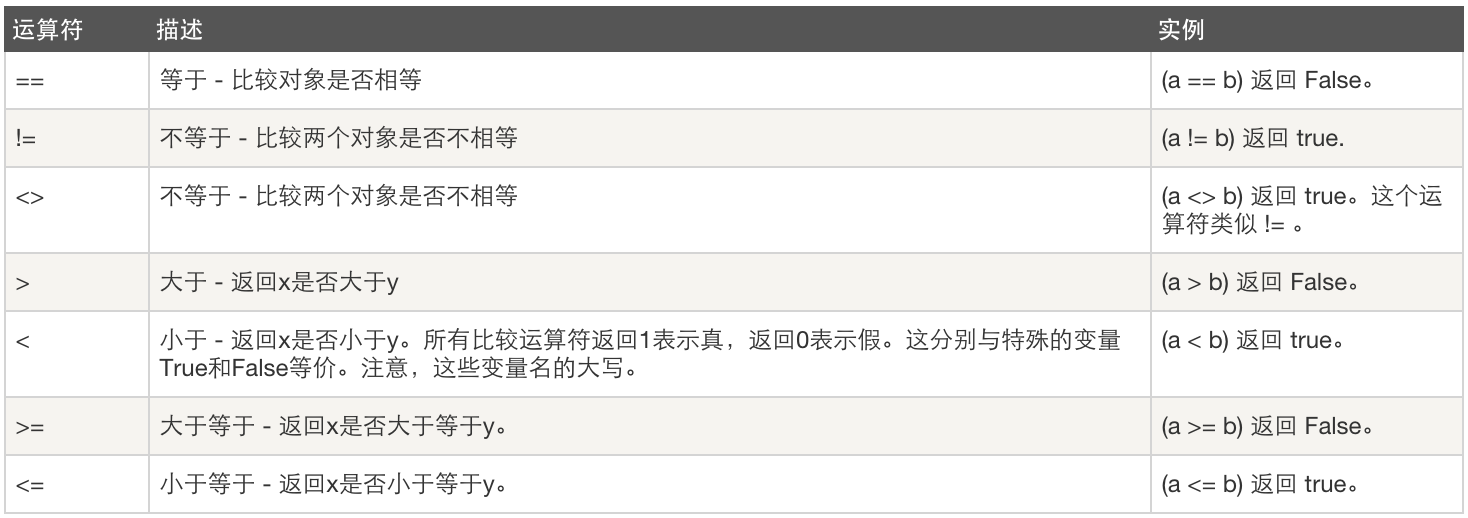三、逻辑运算

以下假设变量：a=10，b=20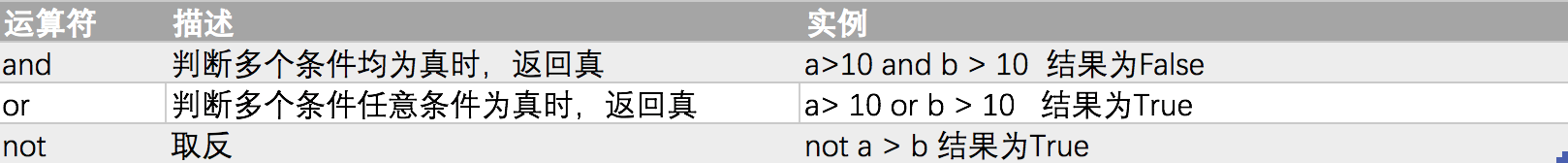四、赋值运算

以下假设变量：a=10，b=20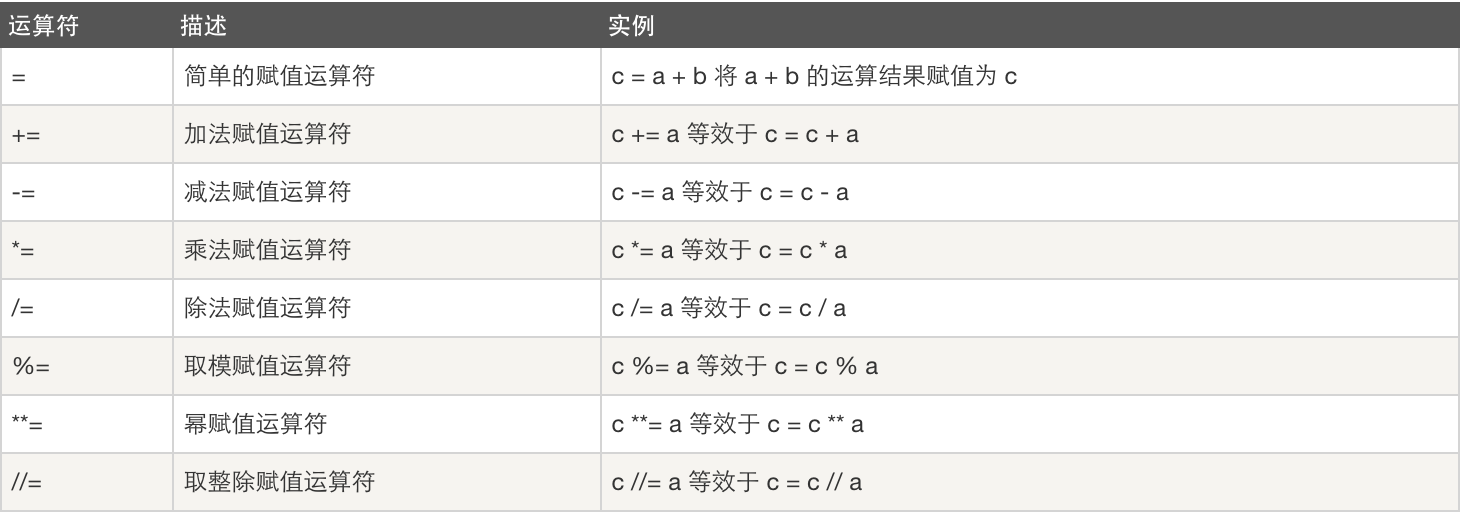五、成员运算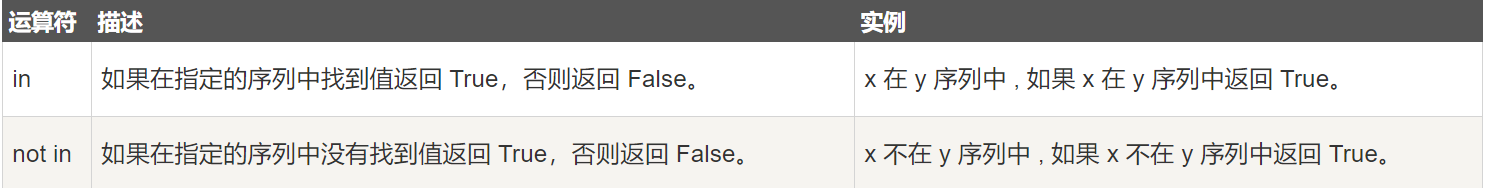示例：

>>> a = "hello"
>>> "he" in a
True
>>> "hl" in a
False
>>> a = [1,5,10,100]
>>> 10 in a
True
>>> 11 in a
False

六、身份运算

== 判断两个变量值是不是相等，is 判断两个变量是不是引用同一个对象，对象id是不是相同。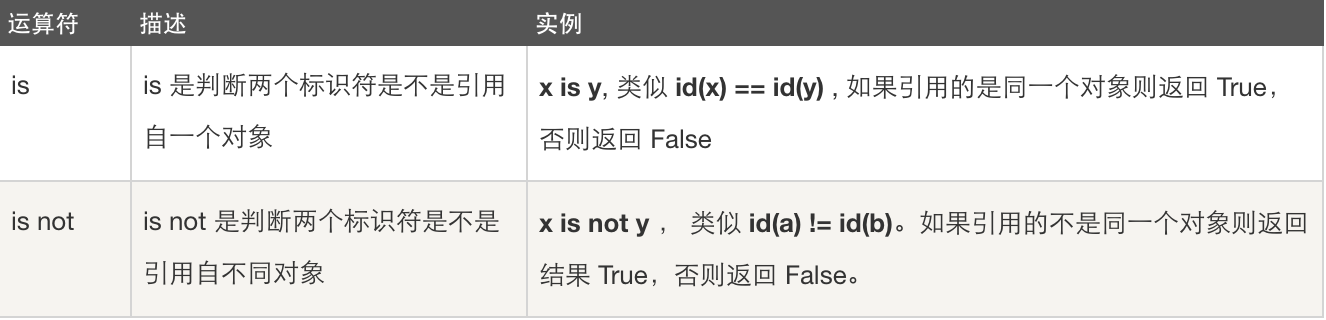之前博客有说，python有内存优化机制，长字符串和[-5,257)范围外的数字没有优化，以此为例，例如：

>>> a = 257
>>> b = 257
>>> id(a)
2588953715824
>>> id(b)
2588983651248
>>> a == b
True
>>> a is b
False
>>> c = 256
>>> d = 256
>>> id(c)
1955897424
>>> id(d)
1955897424
>>> c == d
True
>>> c is d
True

七、位运算

之前博客有说二进制和二进制与十进制的转换，位运算符是把数字看作二进制来进行计算的。

以下假设变量：a=60，b=13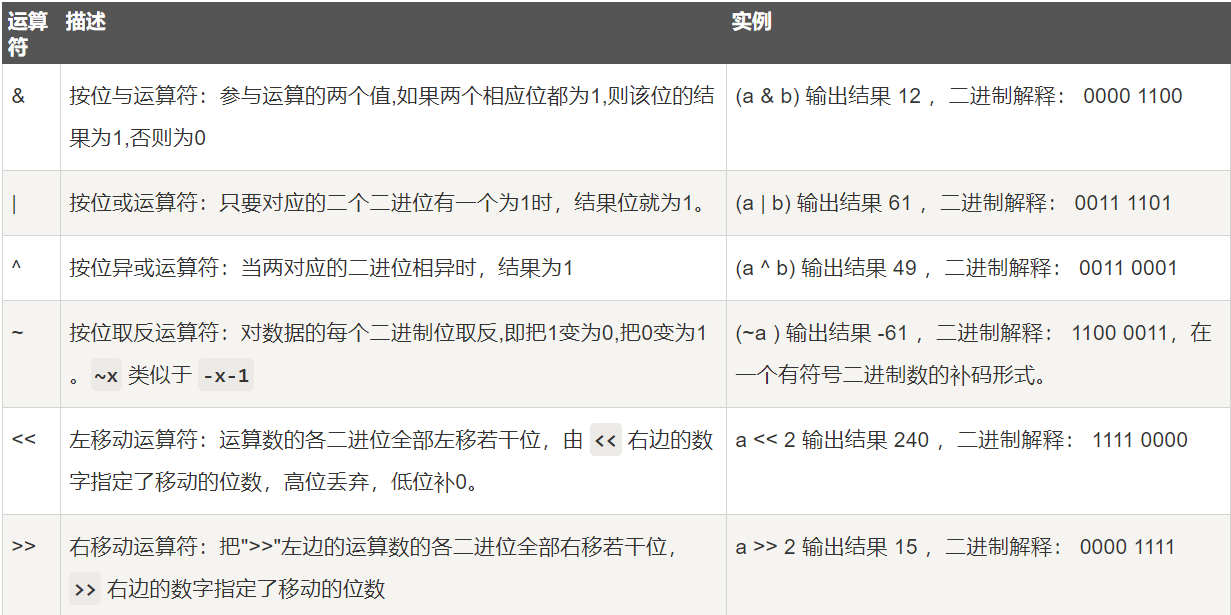# 以下为示例代码，二进制有补0和空格
>>> a = 60
>>> b = 13

>>> bin(a)
'0b0011 1100'
>>> bin(b)
'0b0000 1101'
>>> a&b
12
>>> bin(12)
'0b0000 1100'
>>> a|b
61
>>> bin(61)
'0b0011 1101'
>>> a^b
49
>>> bin(49)
'0b0011 0001'
>>> ~a
-61
>>> bin(-16)
'-0b0001 0000'
>>> a<<2
240
>>> bin(240)
'0b1111 0000'
>>> a>>2
15
>>> bin(15)
'0b0000 1111'

具体的运算符的应用上面已经做了详细说明，下面讲一下这些运算符的优先级，在我们使用的时候，该如何选择合适的运算符，并获取准确的运算结果，这就需要我们对优先级有所了解。

以下表格列出了从最高到最低优先级的所有运算符：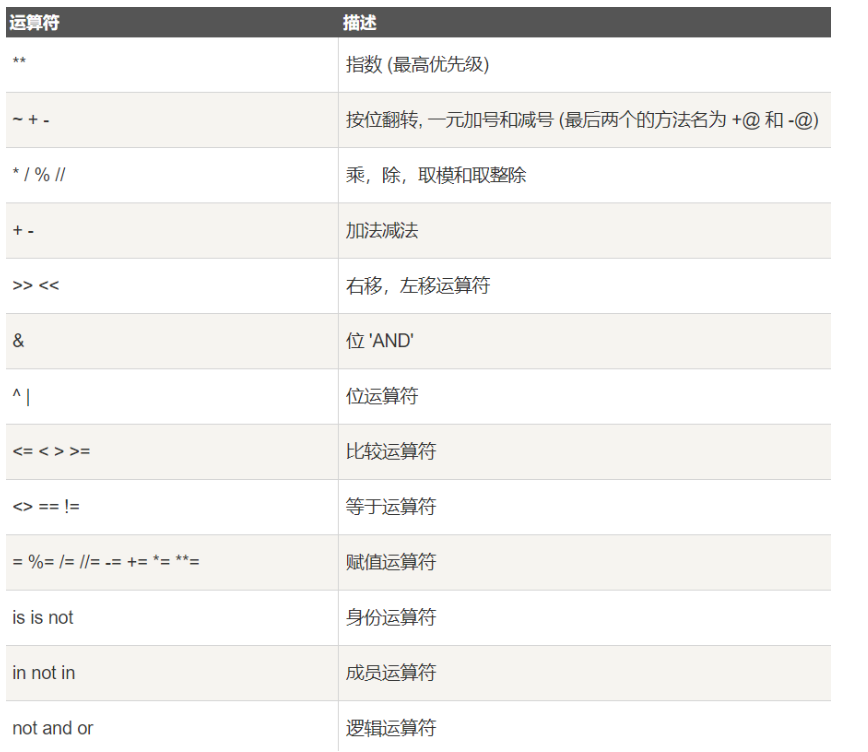展开全文• ## Python——运算符及其优先级

万次阅读 多人点赞 2018-08-02 16:58:17
Python 中，支持以下类型的运算符： 算术运算符 比较（关系）运算符 逻辑（布尔）运算符运算符 赋值运算符 成员运算符 身份运算符 1. 算术运算符 运算符 含义 示例 + 加：两个对象相加，...
在 Python 中，支持以下类型的运算符：

算术运算符
比较（关系）运算符
逻辑（布尔）运算符
位运算符
赋值运算符
成员运算符
身份运算符
1. 算术运算符

运算符
含义
示例
+
加：两个对象相加，或者一元加
x + y
-
减：两个对象相减，或得到负数
x - y
*
乘：两个操作数相乘，或是返回一个被重复若干次的字符串
x * y
/
除：两个操作数相除（总是浮点数）
x / y
%
取模：返回除法（/）的余数
x % y（x/y 的余数）
//
取整除（地板除）：返回商的整数部分
x // y
**
幂：返回 x 的 y 次幂
x ** y
>>> a=10
>>> b=5
>>> print(a+b)
15
>>> print(a-b)
5
>>> print(a*b)
50
>>> print(a/b)
2.0
>>> print(a**b)
100000
>>> print(a//b)
2
>>> print(a%b)
0

2. 比较运算符

运算符
含义
示例
>
大于：如果左操作数大于右操作数，则为 True
x > y
<
小于：如果左操作数小于右操作数，则为 True
x < y
==
等于：如果两个操作数相等，则为 True
x == y
!=
不等于：如果两个操作数不相等，则为 True
x != y
>=
大于等于：如果左操作数大于或等于右操作数，则为 True
x >= y
<=
小于等于：如果左操作数小于或等于右操作数，则为 True
x <= y

>>> a=10
>>> b=20
>>> a==b
False
>>> a!=b
True
>>> a>b
False
>>> a<b
True
>>> a>=b
False
>>> a<=b
True

【注】在一些地方，回用 1 代表 True、0 代表 False。

3. 赋值运算符

运算符
示例
等价于
=
x = 5
x = 5（相同）
+=
x += 5
x = x + 5
-=
x -= 5
x = x - 5
*=
x *= 5
x = x * 5
/=
x /= 5
x = x / 5
%=
x %= 5
x = x % 5
//=
x //= 5
x = x // 5
**=
x **= 5
x = x ** 5
&=
x &= 5
x = x & 5
|=
x |= 5
x = x | 5
^=
x ^= 5
x = x ^ 5
>>=
x >>= 5
x = x >> 5
<<=
x <<= 5
x = x << 5
>>> a=10
>>> b=20
>>> c=0
>>> c=a+b
>>> print(c)
30
>>> c+=10
>>> print(c)
40
>>> c-=a
>>> print(c)
30
>>> c*=a
>>> print(c)
300
>>> c/=a
>>> print(c)
30.0
>>> c%=a
>>> print(c)
0.0
>>> c=a**5
>>> print(c)
100000
>>> c//=b
>>> print(c)
5000
>>> print(b)
20

4. 位运算符

运算符
含义
示例
&
按位与（AND）：参与运算的两个值的两个相应位都为1，则该位的结果为1；否则为0
x & y
|
按位或（OR）：参与运算的两个值的两个相应位有一个为1，则该位的结果为1；否则为0
x | y
~
按位翻转/取反（NOT）：对数据的每个二进制位取反，即把 1 变为 0 ，把 0 变为 1
~x
^
按位异或（XOR）：当两个对应的二进制位相异时，结果为1
x ^ y
>>
按位右移 ：运算数的各个二进制位全部右移若干位
x >> 2
<<
按位左移：运算数的各个二进制位全部左移若干位，高位丢弃，地位不补 0
x << 2

>>> a=10           #a=0000 1010
>>> b=8            #b=0000 1000
>>> a & b          #0000 1000
8
>>> a | b          #0000 1010
10
>>> a ^ b          #0000 0010
2
>>> c=~a           #a的补码 = 原码 = 0000 1010  （0000 1010）取反 （1111 0101）
>>> print(c)       #（1111 0101）取反（0000 1010）
-11                #（0000 1010）+ (0000 0001) = (0000 1011) （前面加上负号）
>>> c=a>>2        #a=0000 1001
>>> print(c)      #c=0000 0010
2
>>> c=a<<2        #c=0010 1000
>>> print(c)
40

【注】~num 单目运算，对数的每一位取反，结果为 -(num+1)

在计算机里面,负数是以补码存储的
原码求补码:取反,+1
补码求原码:取反,+1
取反操作是在原码上进行的！
【例】(1) 求~4, 我们用二进制来表示4:
4 的原码: 0000 0100     取反得到: 1111 1011, 观察符号,是负数,因为负数以补码存储的,所以问题转化为:
某个数 x 的补码是 1111 1011,求 x 的值(由补码求原码)
取反: 0000 0100
+1: 0000 0101 = 5, 加上标点符号(负号) 得到结果: -5

(2) 求 ~-5,同理用二进制表示-5:
因为 -5 是负数,所以它是以 5 的补码表示的,所以转化为已知 5 的补码,求对应的原码,然后在取反.
5补码: 0000 0101,
取反: 1111 1010
+1: 1111 1011, 得到原码 取反: 0000 0100 = 4 ,加上标点负号(正号)得到结果:4

5. 逻辑运算符

运算符
含义
示例
and
x and y
逻辑与：如果 x 为 False，返回 false；否则返回 y 的计算值
or
x or y
逻辑或：如果 x 是非 0，返回 x 的值；否则返回 y 的计算值
not
not x
逻辑非：如果 x 为 False，返回 True；如果 x 为 True，返回 False
>>> a=10
>>> b=20
>>> a and b
20
>>> a or b
10
>>> not a
False
>>> not -1
False
>>> not 0
True

6. 成员运算符

运算符
含义
示例
in
如果在指定序列中找到值/变量，返回 True；否则返回 False
5 in x
not in
如果在指定序列中没有找到值/变量，返回 True；否则返回 False
5 not in x
>>> a=10
>>> b=5
>>> list=[1,2,3,4,5]
>>> a in list
False
>>> a not in list
True
>>> b in list
True
>>> b not in list
False

7. 身份运算符

身份运算符用于检查两个值（或变量）是否位于存储器的同一部分。

注意： 两个变量相等，并不意味着它们也相同。

运算符
含义
示例
is
如果操作数相同，则为 True（引用同一个对象）
x is True
is not
如果操作数不相同，则为 True（引用不同的对象）
x is not True
>>> a= 10
>>> b= 10
>>> print(a is b)
True
>>> print(a is not b)
False
>>> b=20
>>> print(a is b)
False
>>> print(a is not b)
True

8. 运算符优先级

【注】从低到高

运算符
描述
lambda
Lambda表达式
or
布尔“或”
and
布尔“与”
not x
布尔“非”
in，not in
成员测试
is，is not
同一性测试
<，<=，>，>=，!=，==
比较
|
按位或
^
按位异或
&
按位与
<<，>>
移位
+，-
加法与减法
*，/，%
乘法、除法与取余
+x，-x
正负号
~x
按位翻转
**
指数
x.attribute
属性参考
x[index]
下标
x[index:index]
寻址段
f(arguments...)
函数调用
(experession,...)
绑定或元组显示
[expression,...]
列表显示
{key:datum,...}
字典显示
'expression,...'
字符串转换

展开全文• python基础 字符串 运算符 1 布尔运算符 布尔运算符分为两种 1. True 为真 还通俗将就是正确 通过 通电等 2. Flase 为假 与True正好相反 表示不真确 不能通过 不同电 True 数值就是 1 False 数值就是0 ...
python基础  运算符 赋值运算符 比较运算符 逻辑运算符 位运算符 运算符的优先级

1、 布尔运算符

布尔运算符分为两种
1. True 为真 还通俗将就是正确 通过 通电等
2. Flase 为假 与True正好相反 表示不真确 不能通过 不同电
True 数值就是 1
False 数值就是0

print(True+ 1 )
print(False+1)

输出
2
1
进程已结束,退出代码0

2、 算数运算符 与赋值运算符

算数用算符是指运算使用的符号

其中算数运算符还可以作为赋值运算符对赋值进行处理
算数运算符分为
1. 赋值运算符 = 比较运算符 ==
2. 加法运算符 + 加法赋值运算符 +=
3. 减法运算符 -  减法赋值运算符 -=
4. 乘法运算符 * 乘法赋值运算符 *=
5. 除法运算符 / 除法赋值运算符 /=
6. 取余运算符 % 取余赋值运算符 %=
7. 乘方运算符  **乘方赋值运算符 **=
8. 取整运算符 // 与取整赋值运算符//=

1. =  赋值运算符与比较运算符==
将一个值 赋予一个变量

num = （3-3）
print num
print(bool(3==3))


输出：
0
True

进程已结束,退出代码0
2. + 加法运算符 与+=加法赋值运算符
加法运算符可以运算数值相加的运算
还可以对两个值与赋值进行拼接
加法赋值运算符可以将两个赋值相加并并赋与前一个赋值

print(1+1) #加法运算符运算
print('帽子' + '围巾') #加法运算符进行拼接
hat = '帽子'
scarf = '围巾'
hat += scarf #加法赋值运算符进行赋值运算
print(hat)


输出：
2
帽子围巾
帽子围巾

进程已结束,退出代码0

3. - 减法运算符 与-=    减法赋值运算符
减法运算符可以运算数值差的运算
减法赋值运算符可以将两个赋值相减并赋与前一个赋值

print(10 - 9) #减法运算符运算10减9的数值
ten = 10 # 赋值ten为数值10
nine = 9 # 赋值 nine 为数值9
ten -= nine # 减法赋值运算符运算赋值内的差并把结果赋值给ten
print(ten)

输出：
1
1

进程已结束,退出代码0

4. * 乘法运算符 与*=乘法赋值运算符
乘法运算符可以运算数值乘积的运算
乘法运算符还可以使字符串数量倍增
乘法赋值运算符可以将两个赋值相乘并赋与前一个赋值

print(10 * 9)  # 乘法法运算符运算10乘以9的数值
print '帽子' * 2 #将字符串帽子倍增
num1 = 10 # 赋值num1为10
num2 = 9  # 赋值num2 为9
num1 *= num2 # 乘法赋值运算符运算赋值num1乘以赋值num2 并把结果赋值给num1
print(num1)

输出：
90
帽子帽子
90

进程已结束,退出代码0**

5. / 除法运算符 与/=除法赋值运算符
除法运算符可以运算数值的商数运算
除法赋值运算符可以将两个赋值的商数并赋与前一个赋值

print(100 / 5)  # 除法运算符运算100除以5的数值
num1 = 100 # 赋值num1为100
num2 = 5  # 赋值num2 为5
num1 /= num2 # 除法赋值运算符运算赋值num1除以mun2并把运算的值的赋值给第一个值
print(num1)

输出：
20.0
20.0

进程已结束,退出代码0

6.% 取余运算符与取余赋值运算符
取余运算符可以运算数值余数
取余赋值运算符可以求多个赋值的余数并赋与前一个赋值

print(10 % 3)  # 取余算符运算10除以3 只求余数
num1 = 10 # 赋值num1为10
num2 = 3  # 赋值num2 为3
num1 %= num2 # 取余赋值运算符运算赋值num1除以mun2并把运算的值的余数赋值给第一个值
print(num1)

输出：
1
1

进程已结束,退出代码0

7. 幂值运算符与幂赋值运算符
幂值运算符可以运算数值的幂次方
幂赋值运算符将前一个赋值的数为幂的底数后一个数为幂的指数进行运算 并将幂值赋值给前一个名

print(10 ** 3)  # 幂值算符运算10的3次方
num1 = 10 # 赋值num1为10
num2 = 3  # 赋值num2 为3
num1 **= num2 # 幂值运算符以第一个值为幂的底数第二个值为幂的指数进行运算并将结果赋值给第一个值
print(num1)

输出：
1000
1000

进程已结束,退出代码0

8 .取整运算符与取整赋值运算符
取整运算符可以运算商数位 不求余数位
取整赋值运算符可以求除赋值数的商数的整数 余数直接舍去

print(10 // 3)  # 取整算符运算10除以3 只求余数
num1 = 10 # 赋值num1为10
num2 = 3  # 赋值num2 为3
num1 //= num2 # 取整值运算符运算赋值num1除以mun2并把运算的值的整数赋值给第一个值
print(num1)

输出：
3
3

进程已结束,退出代码0

3、比较运算符

比较运算符是用于值与值作为比较使用的

1 . < 小于用于比较前一个数值是不是小于不等于后一个数值如果小于输出为True 大于或等于输出就为False

print(bool(1 < 2))
print(bool(1 < 1))
print(bool(1 < 0))

输出：
True
False
False
进程已结束,退出代码0

2 . > 大于用于比较前一个数值是不是大于不等于后一个数值如果大于输出为True 小于或等于输出就为False

print(bool(2 > 1))
print(bool(2 > 2))
print(bool(2 > 3))

输出：
True
False
False

进程已结束,退出代码0

3 . >=  大于等于用于比较前一个数值是不是大于或等于后一个数值如果大于或者等于输出为True 小于输出就为False否

print(bool(2 >= 1))
print(bool(2 >= 2))
print(bool(2 >= 3))


输出：
True
True
False

进程已结束,退出代码0

4 . <=  小于等于用于比较前一个数值是不是小于或等于后一个数值如果小于或者等于输出为True 大于输出就为False否

print(bool(1 <= 1))
print(bool(1 <= 2))
print(bool(1 <= 0))

输出：
True
True
False

进程已结束,退出代码0

5.== 用于比较对象是否相等 如果等于输出为True 不等于输出就为False

print(bool(1 == 1))
print(bool(1 == 2))


输出：
True
False
进程已结束,退出代码0

6.!= 用于比较对象是不是不等于 如果不等于输出为True 等于就为False

print(bool(1 != 2))
print(bool(1 != 1))

输出：
True
False

进程已结束,退出代码0

4、逻辑运算符

逻辑运算符是对bool值进行运算的运算符

1 .布尔逻辑运算符
and 与 or
and表示逻辑与
or表示逻辑或
运算规则将and看作乘法or看作加法
将True看作1
将False看作0
运算结果为0就是False 否则就是True

a = True   #a等于通过 等于1
b = False   #b等于不通过 等于0
c = a and b #c等于a与b 等于a乘b 等于0
d = a or b  #d等于a或b 等于a+b 等于1
print(bool(c)) #等于0
print(bool(d))  #等于1

输出：
False
True

进程已结束,退出代码0

2 . 成员逻辑运算符
1 . in与not in
in指在里面
not in 指不在里面
例如

a = [1,2,3,4,5,6,7] # 设置列表a存入1234567
b = 8 设置变量b存入8
print(bool(b in a))  #打印布尔值b是不是存在变量a中
print(bool(b not in a))  #打印布尔值b是不是不存在变量a中

输出：
False
True

进程已结束,退出代码0
3 . 身份逻辑运算符
1.测试两个变量是不是同一个ID的运算方法
is
not is
is 与 == 的区别是is确定内容一样ID也必须一样 ==确定的是赋值的内容一样

a = 8  #赋值a为8
b = 8  #赋值b为8
c = ('伊凡博客') #  赋值c为字符串 伊凡博客
d = ('伊凡博客') #  赋值d为字符串 伊凡博客
print(bool(a is b))  #  打印布尔值 a是不是b
print(bool(c is not d))  #打印布尔值 c是不是不是d
print(id(a))  # 打印a的id位置
print(id(b))  # 打印b的id位置
print(id(c))  # 打印c的id位置
print(id(d))  # 打印D的id位置

输出：
True
False
140710419551488
140710419551488
1832655577808
1832655577808

5、Python位运算符

按位运算符是把数字看作二进制来进行计算的。
1. &    按位与运算符：参与运算的两个值,如果两个相应位都为1,则该位的结果为1,否则为0
2. |    按位或运算符：只要对应的二个二进位有一个为1时，结果位就为1。
3. ^    按位异或运算符：当两对应的二进位相异时，结果为1
4. ~   按位取反运算符：对数据的每个二进制位取反,即把1变为0,把0变为1 。
5. <<   左移动运算符：运算数的各二进位全部左移若干位，由 << 右边的数字指定了移动的位数，高位丢弃，低位补0。
6. >>   右移动运算符：把”>>”左边的运算数的各二进位全部右移若干位，>> 右边的数字指定了移动的位数

6 、运算符的优先级

以下表格列出了从最高到最低优先级的所有运算符：

1   **  指数 (最高优先级)
2   ~ + -  按位翻转, 一元加号和减号
3   * / % //    乘，除，取模和取整除
4   + - 加法减法
5   >> <<   右移，左移运算符
6   &   位 ‘and’
7   ^   位运算符
8   <= , < , > ,>=  比较运算符
9   == , != 等于运算符
10  = , %= , /= , //= , -= , += , = , *=  赋值运算符
11  is , is not 身份运算符
12  in , not in 成员运算符
13  not or and  逻辑运算符
展开全文• 比较运算符 赋值运算符 位运算符 逻辑运算符 成员运算符 #in运算符 arr=['user1','user2','user3']; print('user10' in arr); #结果为False 引用运算符 id()该函数可以确定变量引用 1.整型、...
数学运算符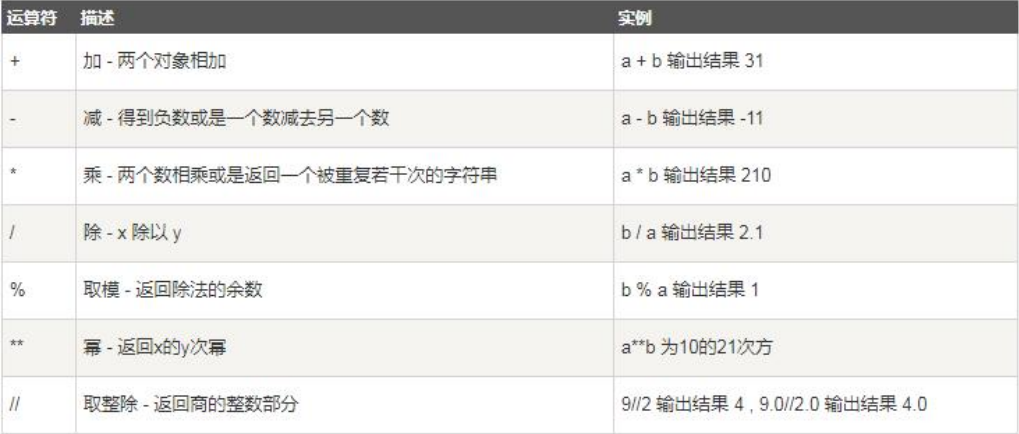比较运算符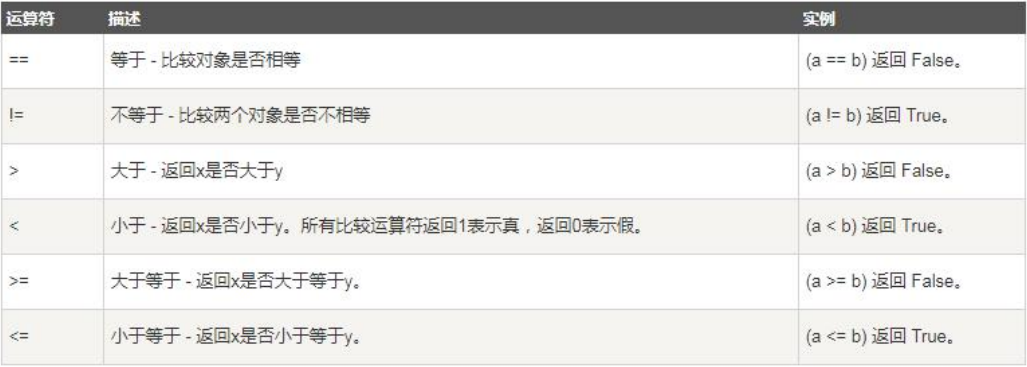赋值运算符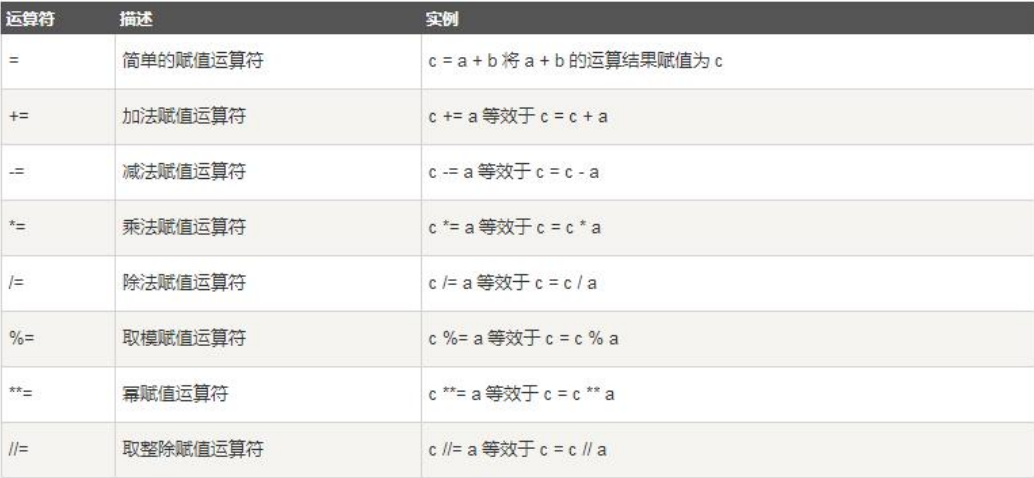位运算符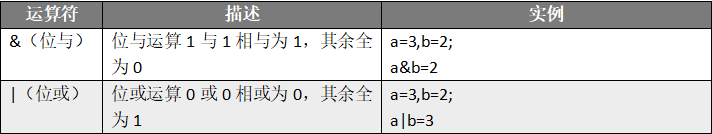逻辑运算符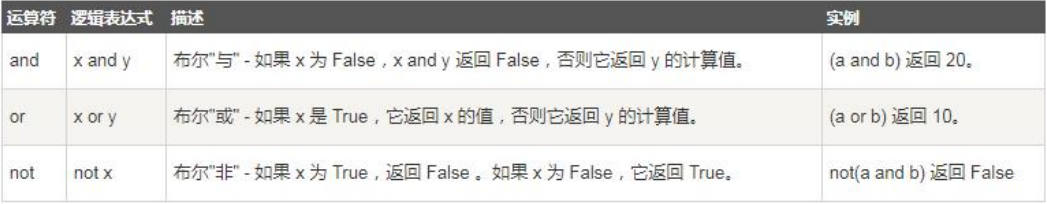成员运算符#in运算符
arr=['user1','user2','user3'];
print('user10' in arr);
#结果为False

引用运算符

id()该函数可以确定变量的引用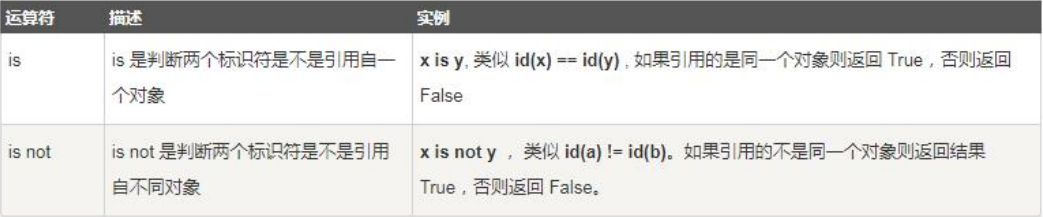1.整型、浮点型、字符串、布尔型、None

1）如果值相同，则使用想让的引用

2）如果值不同，则使用不同的引用

a=10
b=20
c=10
print(id(a))#140708857878464
print(id(b))#140708857878784
print(id(c))#140708857878464
print(a is not c)#False
print(a is not b)#True

2.列表、元组、集合、字典、对象

1）如果两个变量的值相同，也会使用不同的引用

2)  如果使用变量赋值，会使用相同的引用

a=[1,3,5]
b=[1,3,5]
print(id(a))#1602765860352
print(id(b))#1602766610880
print(a is b)#False
a=b
print(id(a))#1602766610880
print(a is b)#True

运算符优先级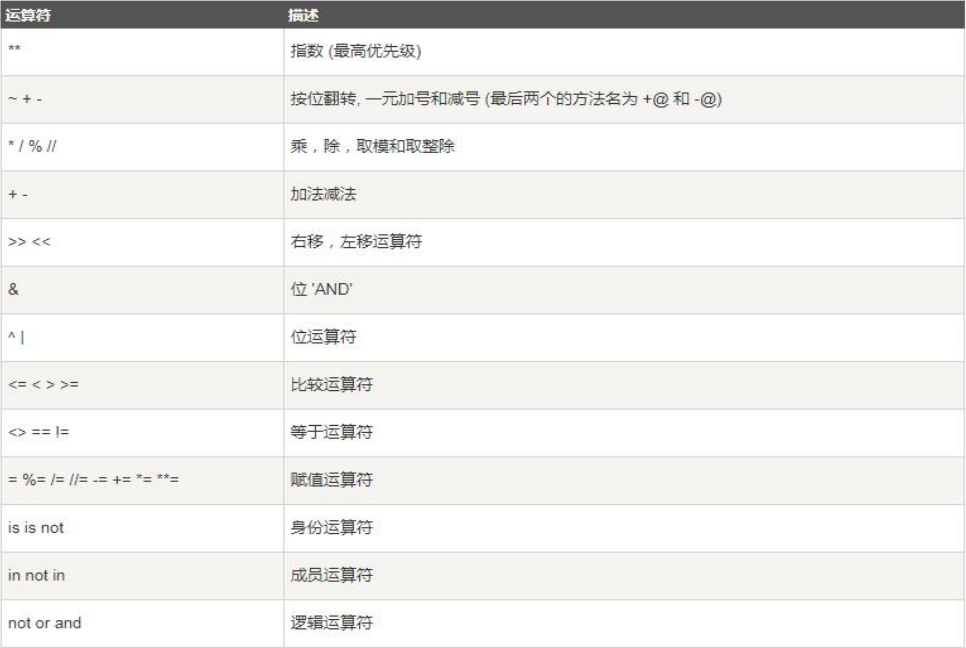print(1+2*3 and 4)#4


展开全文• 一、运算 1、算数运算符（7种） + - * / % (取余数，注意负数取余，要根据除数正负，来套用公式判断余数是...2、比较运算符(6种) == （=是赋值；==是比较） ！= > < >= <= 3、赋值运算符 = += ...
• Python运算符的优先级 运算符 描述 ** 幂（最高优先级） ~ 按位取反 *、/、% 、// 乘、除、取余、取整 +、- 加、减 << 、>> 按位左移、右移 & 按位与 |、^ 按位或、异或 >...
• Python运算符的优先级 优先级高的先运算，优先级低的后运算 运算符 描述 ** 指数（最高优先级） ~、+、- 逻辑非、正数、负数 *、/、%、// 乘、除、取模、取整 +、- 加、减 >>、<< 右移...
• python的运算符包括算术运算符、赋值运算符、比较运算符、布尔运算符、位运算符。 算术运算符：+，-，*，/，//（整除），%（取余），**（幂运算）。 # 注意，一正一负时计算结果会不同 print(4%3) # 1，取余 print...
• 运算符的优先级 运算符 描述 ** 幂 (最高优先级) * / % // 乘、除、取余数、取整除 + - 加法、减法 <= < > >= 比较运算符 == != 等于运算符 not or and 逻辑运算符 = %= /= //= -= ...
• Python运算符优先级表 自上向下，优先级越来越高 运算符描述 lambda Lambda表达式 or 布尔“或” and 布尔“与” not x 布尔“非” in，not in 成员测试 is，is not 同一性......

# python比较运算符的优先级python 订阅